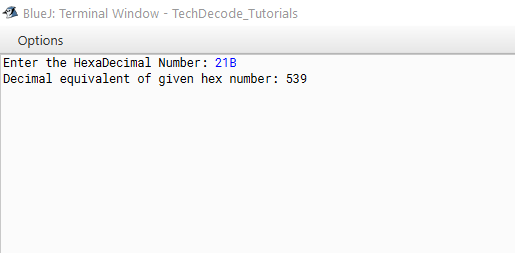# How to convert Hex to Decimal in Java

Java is an `object-oriented programming language` with a high level of abstraction. It is a` general-purpose programming` language designed to allow programmers to write code once and execute it anywhere.  This means that compiled Java code can run on any platform that supports Java without requiring a recompilation.

Today let us know How to convert Hex to Decimal in Java. Decimal is a term that describes the base-10 number system, probably the most commonly used number system. The decimal number system consists of ten single-digit numbers: 0, 1, 2, 3, 4, 5, 6, 7, 8, and 9. The number after 9 is 10. Hexadecimal is the name of the base-16 numeral system. As a result, the numbers 0, 1, 2, 3, 4, 5, 6, 7, 8, 9, 10, 11, 12, 13, 14, and 15 are used in this system. To exist in this numbering system, two-digit decimal numerals 10, 11, 12, 13, 14, and 15 must be represented by a single numeral. The alphabetic letters A, B, C, D, E, and F are used to represent the two-digit decimal values in hexadecimal and are considered legitimate numbers.

## What’s the Approach?

• Import `java.util` package into the class

• Create a Scanner Class Object using `Scanner sc=new Scanner(System.in);`

• Accept the user’s input and store it in a variable named `hex`.

• Convert it to decimal using `Integer.parseInt(hex,16).`

• Print the `Decimal` value.

Also Read: How to convert Long to Int in Java.

### Java Program to convert Hex to Decimal

```/*

* TechDecode Tutorials

*

* How to Convert Hexadecimal to Decimal

*

*/

import java.util.*;

public class Hex_To_Dec

{

public static void main(String args[])

{

//Creating Scanner Class

Scanner sc=new Scanner(System.in);

//Accpeting users input

String hex = sc.nextLine();

hex=hex.toUpperCase();

//converting hex to decimal by passing base 16

int num = Integer.parseInt(hex,16);

System.out.println("Decimal equivalent of given hex number: "+num);

}

}```

Output: